12-240/Classnotes for Tuesday October 16

 Dror's notes above / Students' notes below

Theorems

1. If G generates, |G|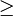$\ge \!\,$ n and G contains a basis, |G|=n then G is a basis

2. If L is linearly independent, |L|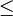$\le \!\,$ n and L can be extended to be a basis. |L|=n => L is a basis.

3.W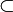$\subset \!\,$ V a subspace then W is finite dimensioned and dim W$\le \!\,$ dim V

If dim W = dim V, then V = W If dim W < dim V, then any basis of W can be extended to be a basis of V

Proof of W is finite dimensioned:

Let L be a linearly independent subset of W which is of maximal size.

2. Bounded :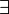$\exist \!\,$ N$\in \!\,$ N,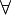$\forall \!\,$ a$\in \!\,$ A, a$\le \!\,$ N
has a maximal element: an element m$\in \!\,$ A,$\forall\!\,$ a$\in \!\,$ A, a$\le \!\,$ m ( m + 1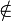$\notin \!\,$ A )